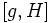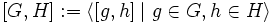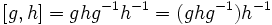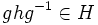# Equivalence of conjugacy and commutator definitions of normality

Jump to: navigation, search
This article gives a proof/explanation of the equivalence of multiple definitions for the term normal subgroup
View a complete list of pages giving proofs of equivalence of definitions

## Statement

Suppose$H$ is a subgroup of a group$G$ and$g \in G$ is an element. Then the following are equivalent:

1.$gHg^{-1} \subseteq H$.
2. The commutator$[g,H] \subseteq H$, where$[g,H]$ is the subgroup generated by$[g,h] = ghg^{-1}h^{-1}, h \in H$.

If these equivalent conditions hold for all$g \in G$, then$H$ is a normal subgroup of$G$. Note that the second condition on all$g \in G$ would translate to saying that$[G,H] := \langle [g,h] \mid g \in G, h \in H\rangle$ is contained in$H$.

Note that$gHg^{-1} \subseteq H$ is a weaker condition than$gHg^{-1} = H$ for a particular$g \in G$.

## More on the proof techniques

The survey article manipulating equations in groups discusses similar proof techniques involving equations that deal with elements and subsets of groups.

## Proof

### (1) implies (2)

Given: Group$G$, subgroup$H$,$g \in G$ such that$gHg^{-1} \subseteq H$.

To prove:$[g,H] \subseteq H$.

Proof: Since$H$ is a subgroup, it suffices to show that all the generators of$[g,H]$ are in$H$, i.e., that$[g,h] \in H$ for every$h \in H$. For this, note that:$\! [g,h] = ghg^{-1}h^{-1} = (ghg^{-1})h^{-1}$

By assumption, since$h \in H$$ghg^{-1} \in H$, and so the quotient$(ghg^{-1})h^{-1}$ is also in$H$. This completes the proof.

### (2) implies (1)

Given: Group$G$, subgroup$H$,$g \in G$ such that$[g,H] \subseteq H$.

To prove:$gHg^{-1} \subseteq H$.

Proof: For any$h \in H$, we have:$\! ghg^{-1} = (ghg^{-1}h^{-1}h = [g,h]h$

By assumption, both$[g,h]$ and$h$ are in$H$, hence$ghg^{-1} \in H$.